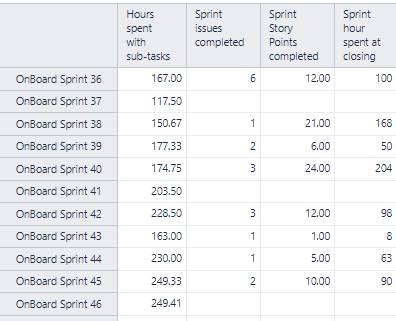# How to sum "Sprint Story Points completed" of 5 rolling latest sprint

Hi,

I wanted to sum the “Sprint Story Points completed” calculated member of the 5 rolling latest sprint but I do not know how to do this.

For example, for Sprint40 I expect 12+0+21+6+24=63, for sprint 41 I expect 0+21+6+24+0 = 51, …Could you help me?
Jérôme

You need the great invention of tuple with that you can use every measure in every way you want to:

([Sprint].CurrentMember.PrevMember,
[Measures].[Sprint Story Points completed])

This gives you the completed story points of the last sprint.

So if you want the last five (including the current), just iterate through them and add them up:

([Sprint].CurrentMember,
[Measures].[Sprint Story Points completed])

([Sprint].CurrentMember.PrevMember,
[Measures].[Sprint Story Points completed])

([Sprint].CurrentMember.PrevMember.PrevMember,
[Measures].[Sprint Story Points completed])

([Sprint].CurrentMember.PrevMember.PrevMember.PrevMember,
[Measures].[Sprint Story Points completed])

([Sprint].CurrentMember.PrevMember.PrevMember.PrevMember.PrevMember,
[Measures].[Sprint Story Points completed])

Maybe there is a more elaborate way but it works.Thanks Sven,

I have a little bit improved the proposed solution

``````Sum(
--set of sprints
{
[Sprint].CurrentMember.PrevMember.PrevMember.PrevMember.PrevMember,
[Sprint].CurrentMember.PrevMember.PrevMember.PrevMember,
[Sprint].CurrentMember.PrevMember.PrevMember,
[Sprint].CurrentMember.PrevMember,
[Sprint].CurrentMember
}
,
--tuple expression for each sprint in a set
(
[Measures].[Sprint Story Points completed]
)
)
``````
1 Like

Hi @J.Bachelet and thanks @sven for the solution!An alternative formula could also be used, however this one will only work with the data visible in the report:

``````Sum(
Tail(